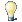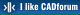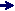#CAD tip # 6381:%  platform  category## Arithmetic functions in AutoCAD fields.In AutoCAD table cells and text fields (texts, block attributes) you can use not only the predefined properties, fields and functions but also the standard mathematic (arithmetic and goniometric) functions with dynamic values.

When defining the arithmetic expressions - see Fields > All > Expression - you can use the following functions:

• +, -, *, /, ^, () = basic arithmetic operations
• sin(angle) = Sinus of an angle
• cos(angle) = Cosinus of an angle
• tang(angle) = Tangens of an angle
• asin(real) = Arcus sinus
• acos(real) = Arcus cosinus
• atan(real) = Arcus tangens
• ln(real) = Natural logarithm
• log(real) = Decimal logarithm
• exp(real) = Natural power
• exp10(real) = Decimal power
• sqr(real) = Square
• sqrt(real) = Square root
• abs(real) = Absolute value
• round(real) = Rounded to the nearest integer
• trunc(real) = Truncated to integer
• r2d(angle) = Angle in radians converted to degrees
• d2r(angle) = Angle in degrees converted to radians
• pi = Pi constant (Ludolf's number)

Example: =atan(pi)

Right-click in the Expression panel of the FIELD dialog and use Insert field... to nest (combine) field expressions and add, subtract, muliply, divide, etc. multiple fields. So you can e.g. multiply the area of the selected closed polyline and its thickness to get the dynamic value of its volume (rt-click, Insert field, object property Area * rt-click, Insert field, object property Thickness). Use "(none)" for formatting the numeric fields.

See video #11

 ACADLT2009ADTCivil*  CAD 10.11.2008    37795×See also: Tip 13583: Evaluate math expressions in AutoCAD DWG texts. Tip 13426: Use of alternative units for arithmetic in dimensions (without square brackets). Tip 12777: Arithmetic and trigonometric functions available in Revit expressions. Tip 12624: How to enter dimensions in Revit using other units? Tip 12002: Arithmetic expressions with measured distances in AutoCAD.

Have we helped you? If you want to support the CAD Forum web service, consider buying one of our CAD applications, or our custom software development offerings, or donating via PayPal (see above). You may also add a link to your web - like this "fan" link:Featuring:Automatic nesting of 2D parts on rectangular sheets in AutoCAD AutoNEST 2008